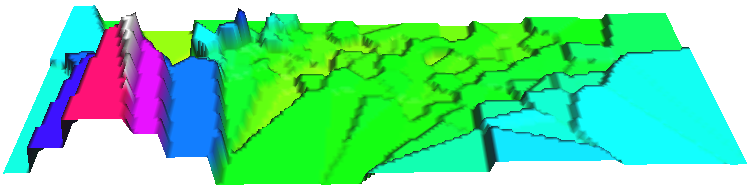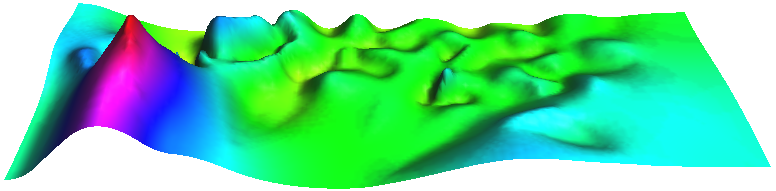## NAME

v.surf.mass - Performs mass-preserving area interpolation.

## KEYWORDS

vector, surface, interpolation, pycnophylactic interpolation

## SYNOPSIS

v.surf.mass
v.surf.mass --help
v.surf.mass [-z] input=name [weight=name] [layer=string] [column=name] output=name [iterations=integer] [threshold=float] [memory=integer] [--overwrite] [--help] [--verbose] [--quiet] [--ui]

### Flags:

-z
Use centroid z coordinates for approximation (3D vector maps only)
--overwrite
Allow output files to overwrite existing files
--help
Print usage summary
--verbose
Verbose module output
--quiet
Quiet module output
--ui
Force launching GUI dialog

### Parameters:

input=name [required]
Name of input vector point map
Or data source for direct OGR access
weight=name
Name of optional weighing raster map
Name of input raster map
layer=string
Layer number or name
Vector features can have category values in different layers. This number determines which layer to use. When used with direct OGR access this is the layer name.
Default: 1
column=name
Name of attribute column with values to approximate
output=name [required]
Name for output raster map
iterations=integer
Maximum number of iterations
Default: 100
threshold=float
Threshold for iterations
Default: 1e-8
memory=integer
Maximum memory to be used for raster output (in MB)
Default: 300

## DESCRIPTION

v.surf.mass creates a raster surface from vector areas, preserving the value of the area attribute. For example, if the selected area attibute is the population count, the sum of all pixel values in a given area is equal to the area's population count.

## NOTES

The current region needs to be prepared with g.region, choosing a resolution such that the smallest area is covered by at least four pixels. The current region should be completely inside the bounding box of the vector.

## EXAMPLE

### Pycnophylactic interpolation of Voronoi triangles using annual precipitation in the North Carolina sample data

(see below for screenshots of the results)
```# setting the region
g.region -p raster=elev_state_500m

# create Voronoi diagram based on meteorological stations
v.voronoi input=precip_30ynormals output=precip_annual

# List of attributes for the vector precip_annual
v.info -c precip_annual

# v.surf.mass converts attributes to density, but rainfall is
# typically measured in mm which is the same for all cells in the
# same input area, thus:
# new column for area size and adjusted precipitation
column="area double precision, prec_adj double precision"
v.to.db map=precip_annual column=area option=area units=meters

# Getting the size of the smallest area
v.db.univar precip_annual column=area

# The smallest area with some population is 1.20789e+08 square meters
# and with a resolution of 5000 meters covered by appr. four pixels
# (depending on the shape of the area). Adjust region for that:
g.region res=5000 -ap

# adjust precipitation values: multiply by area size, dived by pixel size
qcolumn="annual * area / 25000000"

# mass-preserving area interpolation

# rasterize Voronoi diagram for comparison
v.to.rast precip_annual out=precip_annual_voronoi type=area use=attr attrcolumn=annual

# verify results
d.mon wx0
d.rast.leg precip_annual_voronoi
d.rast.leg precip_annual_pycno
```Annual precipitation (30 years avg.) of North Carolina shown as Voronoi diagram based on meteorological stations (perspective view in NVIZ).Smooth Pycnophylactic Interpolation of annual precipitation (30 years avg.) of North Carolina using the Voronoi diagram map based on meteorological stations (perspective view in NVIZ).

## REFERENCES

Tobler WR. 1979. Smooth Pycnophylactic Interpolation for Geographical Regions. Journal of the American Statistical Association, 74 (367): 519-530.

Markus Metz

## SOURCE CODE

Available at: v.surf.mass source code (history)

© 2003-2019 GRASS Development Team, GRASS GIS 7.8.2dev Reference Manual